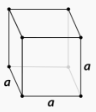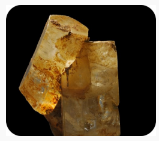# 13.14: Crystal Systems

The development of modern lasers has opened many doors to both research and applications. A laser beam was used to measure the distance from the Earth to the moon. Lasers are important components of CD players. Lasers can provide precise focusing of beams to selectively destroy cancer cells in patients. The ability of a laser to focus precisely is due to high-quality crystals that help give rise to the laser beam. A variety of techniques are used to manufacture pure crystals for use in lasers.

## Crystalline Solids

The majority of solids are crystalline in nature. A crystal is a substance in which the particles are arranged in an orderly, repeating, three-dimensional pattern. Particles of a solid crystal may be ions, atoms or molecules, depending on the type of substance. The three-dimensional arrangement of a solid crystal is referred to as the crystal lattice. Different arrangements of the particles within a crystal cause them to adopt several different shapes.

## Crystal Systems

Crystals are classified into general categories based on their shapes. A crystal is defined by its faces, which intersect with one another at specific angles, which are characteristic of the given substance. The seven crystal systems are shown below, along with an example of each. The edge lengths of a crystal are represented by the letters $$a$$, $$b$$, and $$c$$. The angles at which the faces intersect are represented by the Greek letters $$\alpha$$, $$\beta$$, and $$\gamma$$. Each of the seven crystal systems differs in terms of the angles between the faces and in the number of edges of equal length on each face.

 Table 13.14.1: Seven Basic Crystal Systems and Examples of Each Crystal System Diagram Example Cubic $$a = b = c$$; $$\alpha = \beta = \gamma = 90^\text{o}$$Pyrite Tetragonal $$a = b \neq c$$; $$\alpha = \beta = \gamma = 90^\text{o}$$Wulfenite Orthorhombic $$a \neq b \neq c$$; $$\alpha = \beta = \gamma = 90^\text{o}$$Aragonite Monoclinic $$a \neq b \neq c$$; $$\alpha \neq 90^\text{o} = \beta = \gamma$$Azurite Rhombohedral $$a = b = c$$; $$\alpha = \beta = \gamma \neq 90^\text{o}$$Calcite Triclinic $$a \neq b \neq c$$; $$\alpha \neq \beta \neq \gamma \neq 90^\text{o}$$Microcline Hexagonal $$a = b \neq c$$; $$\alpha = \beta = 90^\text{o}$$, $$\gamma = 120^\text{o}$$Beryl

## Summary

• A crystal is a substance in which the particles are arranged in an orderly, repeating, three-dimensional pattern.
• The crystal lattice is the three-dimensional arrangement of a solid crystal.

## Contributors

• CK-12 Foundation by Sharon Bewick, Richard Parsons, Therese Forsythe, Shonna Robinson, and Jean Dupon.